# ISEE Upper Level Quantitative : How to add variables

## Example Questions

← Previous 1

### Example Question #1 : How To Add Variables

Simplify: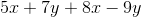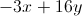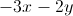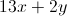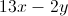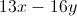Explanation:

Group and collect like terms: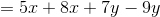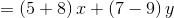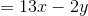### Example Question #2 : How To Add Variables

Simplify the expression: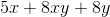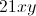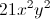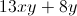The expression cannot be simplified further.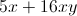The expression cannot be simplified further.

Explanation:

There are no like terms among these three; one has onlyas a variable, one has only, one has both. Therefore, no terms can be collected, and the expression cannot be simplified further.

### Example Question #3 : How To Add Variables

Which is the greater quantity?

(a) The sum of the integers from 1 to 1,000

(b) The sum of the integers from 1,001 to 1,500

It is impossible to tell from the information given

(a) is greater

(a) and (b) are equal

(b) is greater

(b) is greater

Explanation:

(a) The sum of the integers from 1 to 1,000 is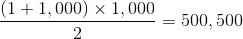(b) The sum of the integers from 1,001 to 1,500 is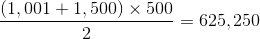(b) is greater

### Example Question #4 : How To Add Variables

Which is the greater quantity?

(A)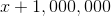(B)It is impossible to determine which is greater from the information given

(B) is greater

(A) is greater

(A) and (B) are equal

It is impossible to determine which is greater from the information given

Explanation:

Which quantity is the greater depends on the value ofCase 1:Then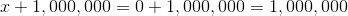and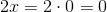Subsequently, (A) is greater

Case 2: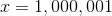Then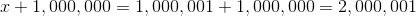and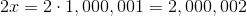Subsequently, (B) is greater

### Example Question #5 : How To Add Variables

Evaluate for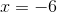: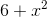Explanation:

Substitute and evaluate, remembering that in order of operations, squaring comes before adding: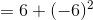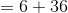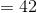### Example Question #6 : How To Add Variables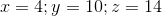Which of the following is the greater quantity?

(A)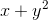(B)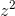(A) is greater

It is impossible to determine which is greater from the information given

(B) is greater

(A) and (B) are equal

(B) is greater

Explanation:

(A) Substitute and evaluate, remembering that by order of operations, squaring precedes addition: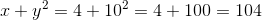(B) Substitute and evaluate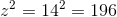(B) is greater

### Example Question #7 : How To Add Variablesis negative. Which of these quantities is the greater?

(A)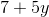(B)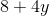It is impossible to determine which is greater from the information given

(B) is greater

(A) is greater

(A) and (B) are equal

(B) is greater

Explanation:

Since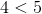andis negative, then by the multiplication property of inequality,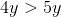Also,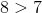, so by the addition property of inequality,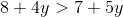making (B) greater.

### Example Question #8 : How To Add Variablesis positive. Which of these quantities is greater?

(A)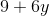(B)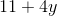It is impossible to determine which is greater from the information given

(A) and (B) are equal

(A) is greater

(B) is greater

It is impossible to determine which is greater from the information given

Explanation:

The values of both terms depend on the value of.

Case 1: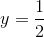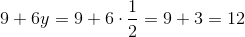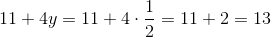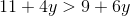, making (B) greater.

Case 2: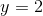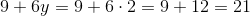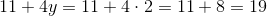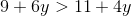, making (A) greater.

Therefore, the information given is insufficient.

### Example Question #9 : How To Add Variables

When evaluating the expression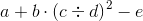,

assuming you know the values of all five variables, what is the third operation that must be performed?

Multiplication

Division

Squaring

Subtraction

Multiplication

Explanation:

The operation that must be performed first is the operation in parentheses, which is the division.

After this, the order of operations is:

Exponents

Multiplication and division (left to right)

Addition and subtraction (left to right)

The second thing that must be done is the exponentiation - the squaring. Of the three remaining operations, the multiplication takes precedence. The correct response is multiplication.

### Example Question #1 : How To Add Variables

The Fibonacci sequence is defined as follows: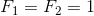For integers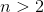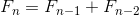.

Which is the greater quantity?

(a) The sum of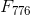and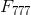(b)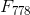It is impossible to determine which is greater from the information given

(a) and (b) are equal

(b) is greater

(a) is greater

(a) and (b) are equal

Explanation:

Using the definition of the sequence for all terms beginning with the third, substitute 778 for: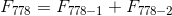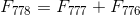The two quantities are equal.

← Previous 1